Last Updated on 16 October, 2021 by Samuelsson

Volume is one piece of information that is often neglected by many market players, especially the beginners. However, learning to interpret volume brings many advantages and could be of tremendous help when it comes to analyzing the markets.

There are many benefits of volume analysis: Firstly, it helps to confirm price trends and chart patterns, as it shows how much interest the security is attracting. Secondly, changes in volume tends to lead the price movements. And thirdly, the trend in a volume indicator over a long period is relevant to the price trends and helps determine when the price is losing momentum.

Experienced traders and investors often use volume analysis to get ahead of others. So we created this guide to teach you what you need to know about volume trading. In this guide, you will learn:

• What volume means
• The common volume indicators
• How to use volume indicators in trading

So let’s begin by having a closer look at what volume is and how it can help traders!

## What Does Volume Mean in the Market?

The market is made up of buyers and sellers; for a transaction to occur, there must be a willing buyer and seller. A unit of volume, therefore, represents a transaction between a buyer and a seller.

The term ‘volume’ means different things for securities that trade in different marketplaces. For forex and other securities that trade over the counter (OTC), the volume might mean the number of ticks (price changes) that occurs in a given time interval. The reason is that there’s no centralized exchange where transactions are recorded. And more importantly, the volume data represents what happens at a particular liquidity provider only.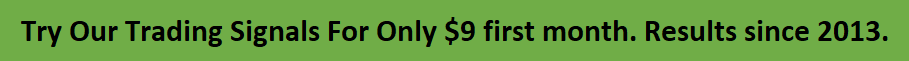For stocks and other securities that trade on standard exchanges, such as futures and options, the volume is a measure of the number of shares or contracts transacted over a specified period of time. In order words, it shows how many times the security has been bought or sold over a given timeframe. The timeframe can be one minute, four hours, one day, or anything.

In most charting platforms, the volume indicator is presented in a separate window below the price chart, just like other indicators used in technical analysis. The volume transacted in the given timeframe is represented as a bar, which can be color-coded.

The color of the bar shows whether the security’s price closes up or down. A green bar is generally used to show that the security closed higher during the trading session while a red bar is used to indicate that the security closed lower. The height of the bar shows whether there’s an increase or a decrease in volume of the security transacted — a taller bar shows a higher volume while a shorter bar shows a lower volume.Significant Volume Spikes

### The Significance of Little Volume

It makes little sense to analyze the volume alone. To correctly interpret the volume data, it must be seen in the light of what the price is doing. So the implication of a small trading volume depends on the price movement.

If the price is rising in an uptrend but the volume is reducing or unchanged, it may show that there’s little interest in the security, and the price may reverse. Similarly, when the price is rapidly declining but the volume is low, it could mean that the institutional traders are not interested in the price direction. So the price will soon reverse to the upside.

### The Significance of High Volume

A high volume usually indicates more interest in the security and the presence of institutional traders. How the volume will affect the price movement depends on the market situation. In an uptrend, an increasing price accompanied by a rising volume may be a sign of a healthy uptrend. In the same way, a declining price in a downtrend occurring with an increasing volume indicates a possible downtrend continuation.

However, for a prolonged uptrend or downtrend, the case is different. A rapidly rising price in an uptrend accompanied by a huge volume may be a sign of exhaustion — a situation known as a buying climax. Similarly, a rapidly declining price in a prolonged downtrend occurring with a huge volume might mean capitulation — a situation where hesitant investors finally give up on the market and massively sell their stocks.

To help traders and investors better interpret the volume of the market, there are many volume-based indicators you could use. Let’s take a look at some of the most common volume indicators around.

## Volume Indicators

Apart from the volume itself, there are a lot of other indicators that are based on the volume data. Analyzing those volume indicators has always helped traders and investors to better understand what is happening in the market. Here are some of the commonly used volume indicators:

• OBV indicator
• Volume RSI
• Volume price trend indicator
• Money flow index
• Chaikin money flow indicator
• Accumulation/distribution
• Ease of movement
• Negative volume index
• Volume-weighted average price

## OBV Indicator

The OBV indicator, popularly known as on-balance volume, is a technical analysis indicator that relates volume flow to changes in a security’s price. It uses a cumulative total of positive and negative trading volume to predict the direction of price. The OBV is a volume-based momentum oscillator, so it is a leading indicator — it changes direction before the price.

This volume indicator was created by Joseph Granville in his 1963 book titled, “Granville’s New Key to Stock Market Profits”. Granville proposed the theory that changes in volume precede price movements in a measurable way. He believed that volume was the main force behind major market moves and thought of OBV’s prediction of price changes as a compressed spring that expands rapidly when released.

He gave the formula OBV as:

OBV = OBVpre +

Where:

OBV = current on-balance volume level

OBVprev = previous on-balance volume level

### How OBV is Calculated

OBV is a running total of the trading volume and shows whether there’s an inflow or outflow of volume. So, depending on how the current price closes relative to the preceding session’s close, there are three ways the current OBV can be calculated:

1. If the current session’s closing price is higher than the preceding session’s closing price, then: Current OBV = Previous OBV + Current session’s volume
2. If the current session’s closing price is equal to the preceding session’s closing price, then: Current OBV = Previous OBV
3. If the current session’s closing price is lower than the preceding session’s closing price — Current OBV = Previous OBV – Current session’s volume

### How to Interpret the OBV

It is believed that the OBV shows the interactions between the institutional and retail traders in the market. The OBV is plotted as a line chart on a separate window from the main price chart. Being a cumulative total volume, the OBV indicator should be going up when the price is going up and be going down when the price is falling.

If the price makes a new high, the OBV should also make a new high. If the OBV makes a lower high when the price makes a higher high, there’s a classical bearish divergence — indicating that only the retail traders are buying. Another type of bearish divergence occurs when the price remains relatively quiet and fails to make a higher high but the OBV soars higher than the previous high — indicating that the institutional traders are accumulating short positions. In both situations, the chances are high that the price will turn downwards.

On the other hand, if the price makes a lower low and the OBV makes a higher low, there is a classical bullish divergence, showing that the institutional traders don’t believe in that move. Also, if the OBV makes a lower low when the price is relatively unchanged or makes a higher low, a non-classical bullish divergence occurs, indicating that the smart money (institutional traders) is accumulating long positions.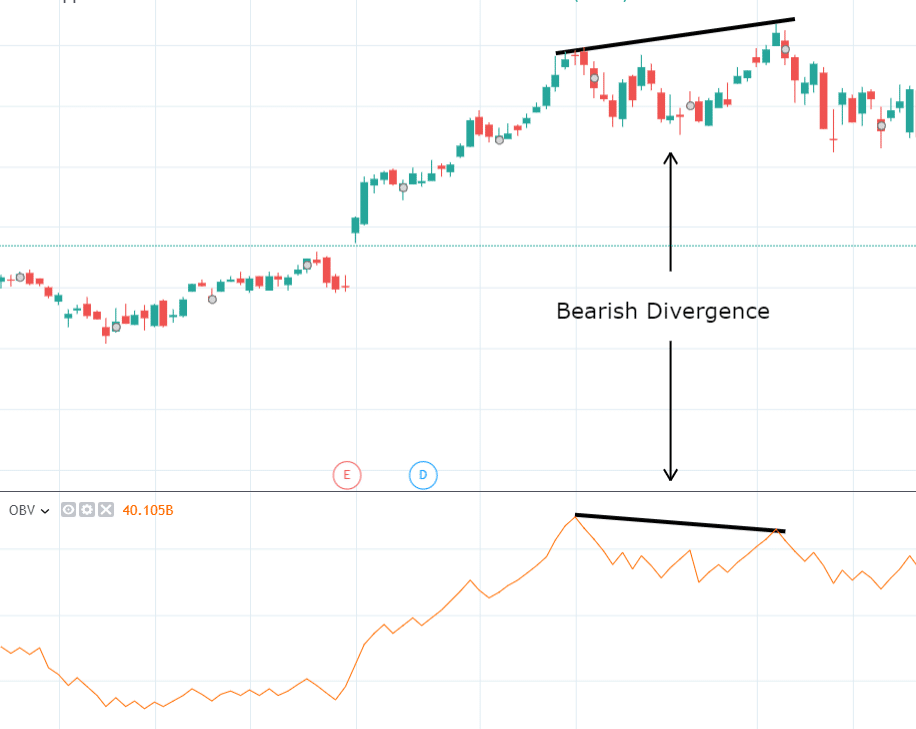OBV – On Balance Volume

## Volume RSI

The volume RSI is a volume indicator that measures the speed and change of volume during the price up-close (up-volume) and during the price down-close (down-volume). It is a momentum indicator that tries to gauge changes in price trend via changes in bullish (when the price closed up) and bearish (when the price closed down) volume data.

In other words, the volume RSI is similar to the price-based RSI, except that changes in volume data are used instead of changes in price. However, the direction of the change (up-volume or down-volume) is determined by the direction of price close. So this volume indicator compares the volume traded during trading sessions when price closed higher to the volume traded when price closed lower to know which is stronger.

### How is Volume RSI Calculated?

Volume RSI is calculated like the price-based RSI, the difference being that volume data is used instead of price data. The calculation follows a four-step process:

1. Calculating the up-close and down-close changes in volume
2. Getting the n-period smoothed moving average of the up-volume and down-volume
3. Calculating the relative strength factor (RS)
4. Calculating the RSI
5. Calculating the up-volume and down-volume:

If price closed up, Up-volume = current volume – previous volume, and Down-volume = 0

If price closed down, Down-volume = previous volume – current volume, and Up-volume = 0

If price closed unchanged, Up-volume = 0, and Down-volume = 0

1. Getting the n-period smoothed moving average (SMA) of the up-volume and down-volume:

The first averages are calculated as follows:

First Average Up-volume = Sum of Up-volumes over the past n periods / n.

First Average Down-volume = Sum of Down-volume over the past n periods / n

The other averages are calculated from the previous averages and the current Up-volume/Down-volume:

Average Up-volume = [(previous Average Up-volume) x (n – 1) + current Up-volume] / n.

Average Down-volume = [(previous Average Down-volume) x (n – 1) + current Down-volume] / n.

1. Calculating the relative strength factor (RS):

The relative strength factor is the ratio of the Up-volume Averages to the Down-volume Averages:

RS = SMMA (Up-volume, n)/SMMA (Down-volume, n)

1. Calculating the RSI:

RSI = 100 – [100/(1 + RS)]

### How to Interpret Volume RSI

The volume RSI is interpreted the same way as the price-based RSI. Just like the price type, the volume indicator oscillates between 0 and 100% and around the 50% line. Below the 30% line is considered oversold while above the 70% line is considered overbought.

When the indicator is rising from the oversold region and crosses the 50% line, bulls are dominating. On the other hand, when the indicator is coming down from the overbought region and crosses the 50%, bears are dominating.

In the image below you can see the same divergence as we showed you with the OBV indicatorVolume RSI

## Volume-Price Trend Indicator (VPT)

Volume-price trend, also called price-volume trend (PVT), is a volume indicator that relates the volume of security transacted with the fractional change in price. It helps to determine both the price direction and the strength of the price move. Thus, the VPT shows the balance between the demand and supply of the asset and how it affects the price.

This volume indicator is a running cumulative value, so it adds or subtracts a product of the current volume and a fractional change in price to the previous VPT value — depending on the direction of the security’s close price — to get the current VPT value.

### How to Calculate the Volume-price Trend Indicator

The volume-price trend indicator is calculated as follows:

VPT = VPTprev + Volume x {(Price Closecurrent – Price Closeprev)/Price Closeprev}

Where:

VPT = Current value of the Volume-price Trend

VPTpre = Previous value of the Volume-price Trend

Volume = Current Session’s Volume

Price Closecurrent = Current Session’s Closing Price

Price Closeprev = Preceding Session’s Closing Price

Just like the on-balance volume, the start point in the indicator is taken arbitrarily, so the actual value of the indicator is not that important — the shape of the line graph is what matters. Unlike the OBV which uses volume alone in accordance with the direction of price close, the VPT factors in the extent of the higher or lower price close.

### What Does the Volume-price Indicator Tell You?

The interpretation of this volume indicator is similar to that of OBV. The main idea is there’s a higher volume when the price is moving in predominant trend direction and less volume when the price is moving against the main trend. So if the main trend is up, the volume is expected to be high for a trading session that closes up, and in a downtrend, the volume should be high for sessions that close lower.

Since the VPT is a cumulative direction-based value, it should be going up when a session is trading up in an uptrend and be going down when a session is trading down in a downtrend. If the price makes a higher high in an uptrend, the VPT should too; if not, a bearish divergence is created which might signal a price reversal. The opposite is the case in a downtrend — if the price makes a lower low, the VPT should also make a lower low, and if it doesn’t, a bullish divergence is formed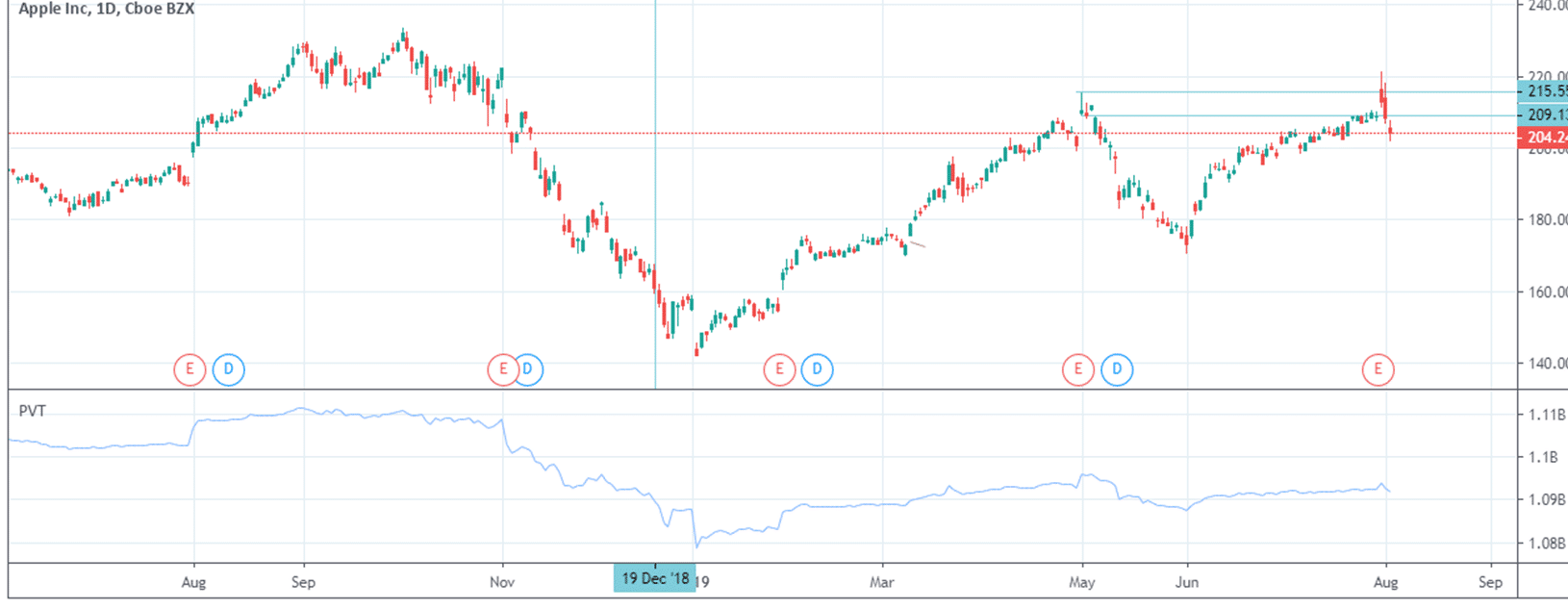volume-price indicator

## Volume Oscillator (VO)

The volume oscillator is a volume indicator that displays the difference between two volume-based moving averages as a percentage of the slower moving average. It consists of two moving averages of the volume data, one fast and the other slow. The difference between the two moving averages is expressed as a percentage of the slower moving average.

In essence, the indicator is similar to the OsMA (oscillatory moving average indicator), except that volume data is used instead of price. Sometimes, chartists add a moving average of the difference to serve as a signal line, thereby making the indicator look more like a volume-based MACD, and calls the indicator percentage volume oscillator (PVO).

### How to Calculate the Volume Oscillator

VO = {(short EMA of volume – long EMA of volume)/long EMA of volume} x 100

Where:

VO = volume oscillator

Short EMA of volume = short-period exponential moving average of volume (usually 12 or 14 periods)

Long EMA of volume = long-period exponential moving average of volume (usually 26 or 28 periods)

In the PVO indicator, a 9-period moving average of the VO is added as a signal line.

### How to Interpret the Volume Oscillator

The VO is usually displayed as a single line that oscillates around the zero line, but it can also be displayed as bars above and below the zero line. When the indicator is at the zero level, it means that the faster moving average is crossing the slower moving average, as the difference between the moving averages will be zero.

When the indicator is rising above the zero line, the faster moving average is rising above the slower moving average — indicating a short-term surge in volume. Falling below the zero line means that the faster moving average is falling below the slower one, showing a decline in volume.

It is expected that strong price moves, up or down, should be accompanied by an increase in volume. So the indicator helps to confirm the force behind price movements. An increase in price in an uptrend or a decrease in price in a downtrend accompanied by a rise in the volume oscillator is a sign of strength in the trend direction. When such moves are accompanied by a decrease in the volume oscillator, there’s a weakness in that trend direction.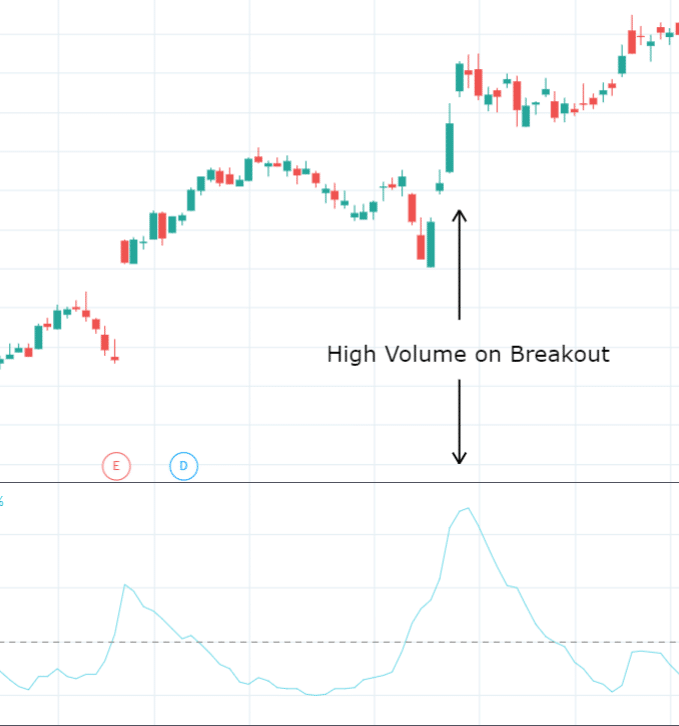Volume Oscillator

## Money Flow Index (MFI)

The money flow index is a volume indicator that oscillates between 0 and 100. It is used to indicate the direction of money flow by estimating the values of recent transactions and the net direction of the transactions. It uses both price and volume data to identify overbought and oversold regions.

This volume indicator was created by Gene Quong and Avrum Soudack. Using the same logic as the relative strength index (RSI), the MFI is often referred to as the volume-weighted RSI by some analysts because it uses both price and volume data in the calculation.### How to Calculate the Money Flow Index

The money flow index is calculated like the RSI, and it follows a four-step process:

1. Calculate the typical price
2. Calculate the positive and negative money flow
3. Calculate  the money flow ratio
4. Calculate  the money flow index
5. Calculate the typical price:

This is the average of the high, low, and close prices of the trading session. The formula is as follows:

Typical price = (high + low + close)/3

1. Calculate the positive and negative money flow:

Money flow = typical price x volume

But you need to get the positive and negative money flows:

• Positive money flow is gotten by adding the money flow of all the sessions where the typical price is greater than the preceding session’s typical price
• Negative money flow is gotten by adding the money flow of all the sessions where the typical price is less than the preceding session’s typical price
• For sessions where the typical price is the same as the preceding session, the money flow is not calculated
1. Calculate the money ratio:

This is a ratio of the positive money flow to the negative money flow, and it’s given as:

Money ratio = positive money flow / negative money flow

1. Calculate the money flow index:

Money flow index (MFI) = 100 – [100/(1 + money ratio)]

### How to Interpret the MFI

The indicator shows how much a security is traded and the percentage that traded to the upside. Generally, a value of 80 and above is considered overbought while 20 and below is considered oversold, but the creators of the indicator recommended 90 and 10 for overbought and oversold levels respectively.

In addition to the overbought and oversold regions, a divergence between the price and the indicator is important. For example, if the price is making a higher high but the indicator is making a lower high, the move is weak, and the price may reverse.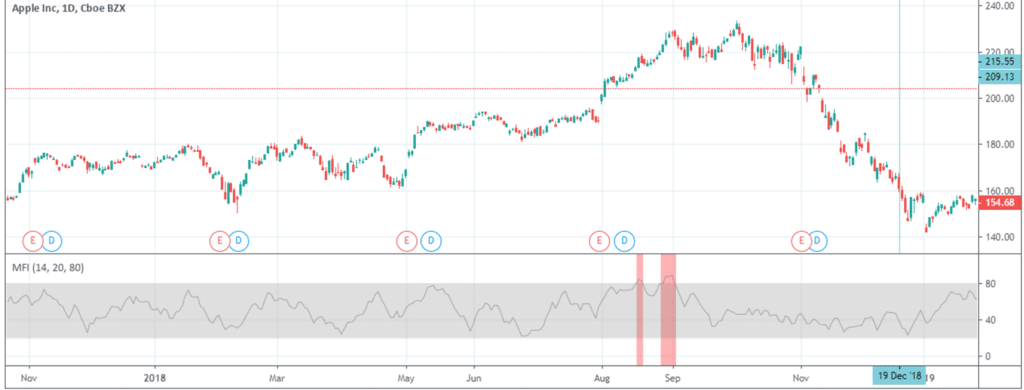Money Flow Index

## Chaikin Money Flow Indicator

The Chaikin money flow indicator (CMF) is a volume indicator that measures the money flow volume over a chosen period, usually 20 or 21 periods. Created by Marc Chaikin, the indicator uses the money flow volume — which also forms the basis for the accumulation distribution index(covered soon) — but instead of a cumulative total, the CMF gets the sum of the money flow volume.

The money flow volume is a measure of the volume and where the price closed relative to the trading session’s range. It comes from the idea that buying pressure is indicated by a rising volume and recurrent closes in the upper part of the session’s price range while selling pressure is demonstrated by an increasing volume and repeated closes in the lower part of the price range.

Both buying and selling pressures are accompanied by an increase in volume, but the location of the closing prices are in accordance with the direction of price. So the indicator oscillates about the zero line — above zero indicating a bullish trend and below, a bearish trend.

### How Chaikin Money Flow is Calculated

Calculating the CMF is a three-step process:

1. Calculating the close location value (CLV), also known as the money flow multiplier
2. Calculating the money flow volume
3. Calculating the CMF

Step 1: Calculate the close location value

This show where the price closed relative to the session’s range. It is gotten as follows:

CLV = [(Close – Low) – (High – Close)] / (High – Low)

Step 2: Calculate the money flow volume

Money flow volume = CLV x Volume

Step 3: Calculate the Chaikin Money Flow

CMF = (n-period sum of Money Flow Volume) / (n-period sum of Volume)

Where:

n is the period of choice, usually 20 or 21.

### How to Interpret the Chaikin Money Flow

This volume indicator oscillates between +1 and -1 but may never reach these extremes as it would take consecutive closing at the upside or downside for the entire n-period for the CMF to get to a +1 or -1 value respectively.  For the most part, it will just oscillate between +0.4 to -0.4, with 0 as the centerline.

When the indicator rises above the zero line into the positive territory, there is a rising buying pressure and waning selling pressure. Conversely, when it descends below the zero line, there is a rising selling pressure and a declining bullish pressure. The indicator can also show bearish and bullish divergences.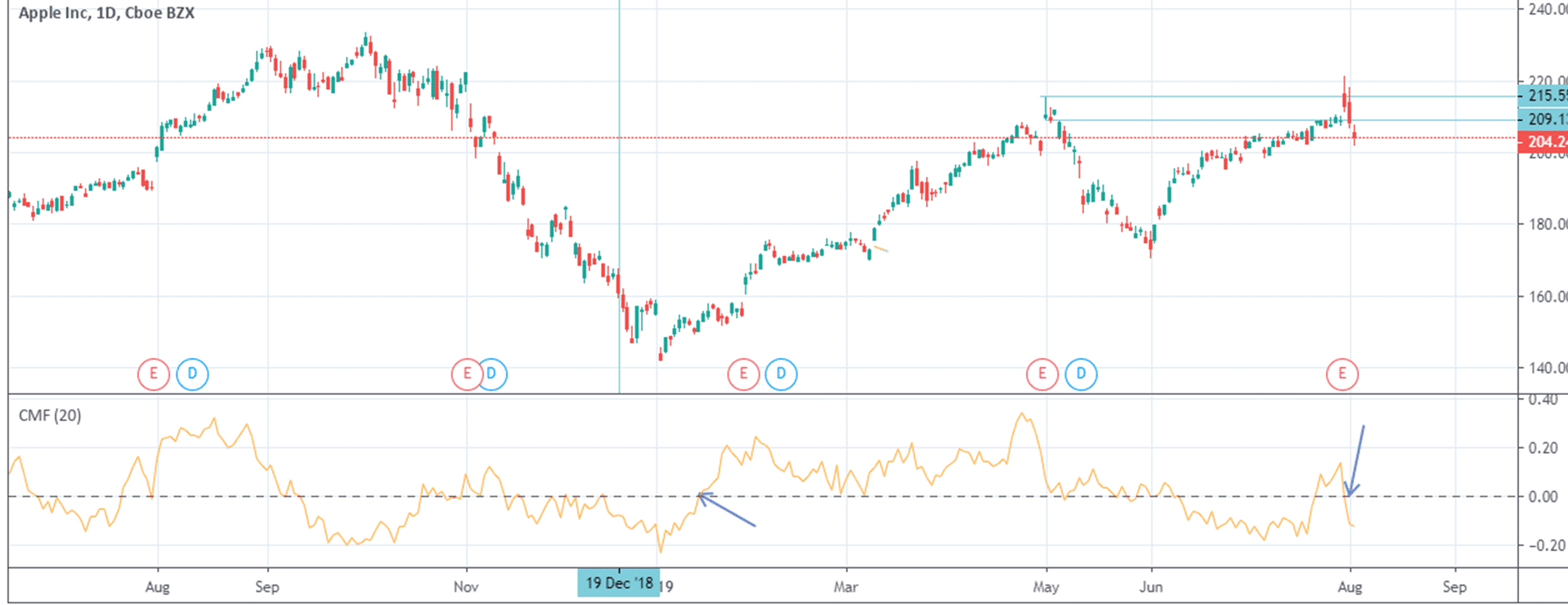Chaikin Money

## Accumulation/Distribution (A/D)

Being a volume indicator, the accumulation/distribution index relates the volume of a security transacted to the changes in its price. It uses volume and price data to determine whether a stock is currently being accumulated or distributed. Because it uses volume to gauge price momentum, it is a leading indicator.

The index tries to assess the demand and supply of the security by checking where the price closed during the session’s range and how much volume was traded in the session. Just like the OBV, the A/D is a running cumulative value in which the current value is added to the previous value to get the current cumulative total.

### How to Calculate the A/D

A/D = Previous A/D + CMFV

Where:

CMFV = Current Money Flow Volume

{[(Close – Low) – (High – Close)] / (High – Low)} x V

To simplify the calculation of the A/D, we need to break it down to three steps:

1. Get the close location value (CLV) — this is also known as the money flow multiplier
2. Calculate the current money flow volume (CMFV)
3. Compute the current A/D value

#### Step 1: Get the Close Location Value

It measures the level of closing price relative to the range.

CLV = [(Close – Low) – (High – Close)] / (High – Low)

#### Step 2: Calculate the Current Money Flow Volume

CMFV = CLV x Volume

#### Step 3: Compute the Current A/D Value

A/D = Previous A/D + CMFV

The starting point in the A/D is arbitrary, so the actual value of the A/D is not that important. It is the shape of the A/D line that matters.

### How to Interpret the A/D Line

The indicator shows how the price closes and the force behind the move. Because it is gotten from the product of the close location and the volume, the indicator rises faster if price closes near the high with a large volume — indicating a strong buying pressure. If, on the other hand, the price closes near the low of the session’s range with a large volume, the A/D line will decline faster, showing a strong selling pressure.

The indicator helps in assessing price trends and potential price reversals. When the price is in an uptrend, the indicator should also be in an uptrend. If the indicator is in a downtrend, it may indicate a selling pressure (distribution), and the price is likely to reverse to the downside. Conversely, if the price is in a downtrend and the A/D line is ascending, it shows there may be a buying pressure (accumulation), and the price may soon rise.Accumulation/Distribution

## Ease of Movement (EOM)

Developed by Richard Arms, the Ease of Movement indicator (also known as the Ease of Movement Value (EMV)) is a volume indicator that tries to use both momentum and volume data to ascertain how easily the price can move in a particular direction. It oscillates above and below the zero line, corresponding to bullish and bearish movements respectively.

In theory, if the price is able to move up easily, there is little selling pressure; thus, it will continue to rise. Similarly, if the price can fall easily, there’s little buying pressure, so it may continue to fall. The EOM is usually seen as a volume-weighted momentum indicator because of the way it is calculated.

### How to calculate the EOM

The EOM formula has three components, namely: distance moved, volume, and the high-low range. The calculation is normally done in four steps:

1. Calculating the distance moved
2. Calculating the box ratio
3. Calculating the 1-period EOM
4. Computing an n-period EOM

Step 1: Calculate the distance moved

This will form the numerator of the EOM and is gotten by comparing the current session’s midpoint with the preceding session’s midpoint, as follows:

Distance Moved = [(Current High + Current Low)/2  –  (Preceding High + Preceding Low)/2]

Step 2: Calculate the box ratio

This involves the volume and the high-low range components of the formula, and it forms the denominator of the EMV. It is gotten as follows:

Box Ratio = [(V/100,000,000) / (Current High – Current Low)]

Step 3: Calculate the 1-period EOM

1-Period EOM = Distance Moved / Box Ratio

Step 4: Compute the n-period EOM

Generally, a 14-period is used.

14-Period EOM = 14-Period Simple Moving average of the 1-Period EOM

### How to Interpret the EOM

The EOM is larger when the denominator, the box ratio, is smaller. And the box ratio is smaller when the volume is relatively low and the high-low range is relatively large. A wide price range on low volume would imply an easy price movement since it didn’t take a large volume to move the price. The opposite is also true when there’s a small price range on high volume, showing difficulty in the price movement.

When the EOM climbs above the zero line and continues rising, it suggests that the price is rising on low volume, so there’s an ease of movement to the upside. Conversely, when the indicator falls below the zero line and keeps descending, there’s an ease of movement to the downside.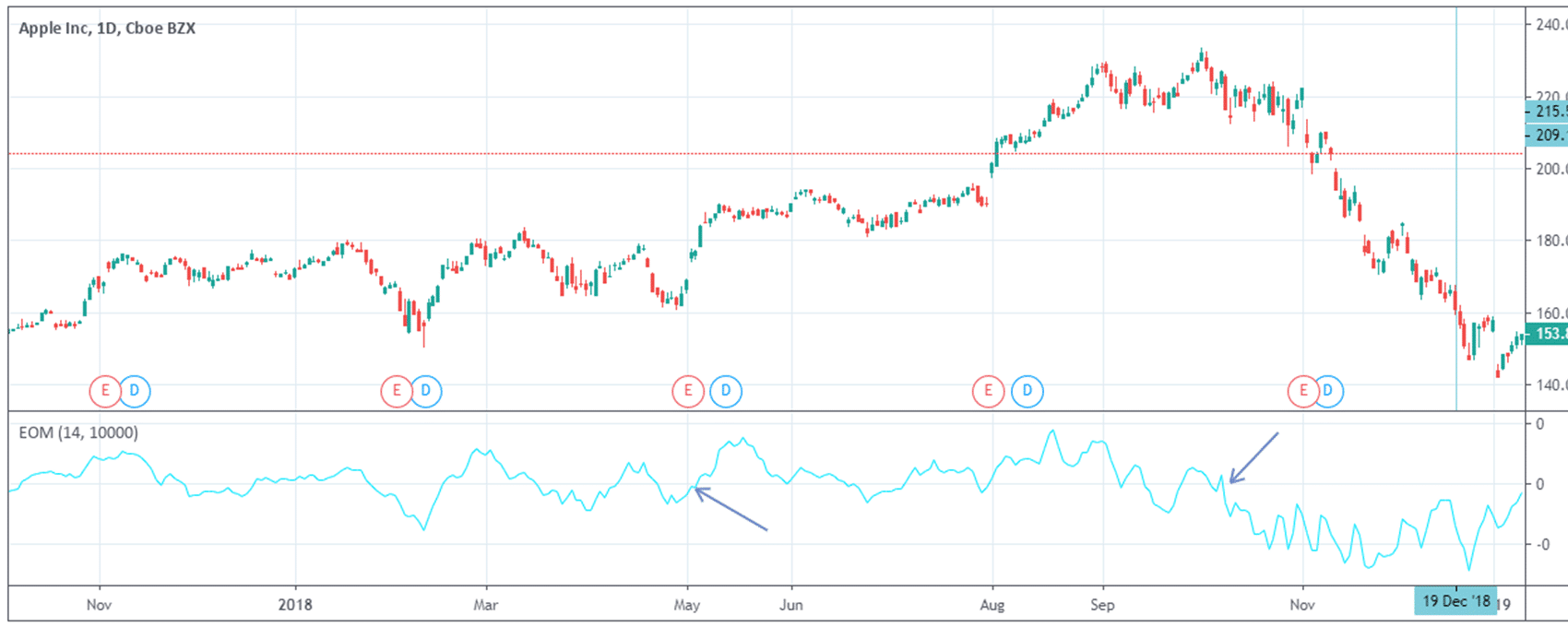Ease of Movement

## Negative Volume Index

This volume indicator combines both volume and price data to graphically show how sessions with low volume can affect price movements. It tries to use the changes in volume to track what the smart money (institutional investors) is doing. It can be used in combination with the positive volume index.

Both indexes were developed in the 1930s by Paul Dysart but weren’t well-known until after his death in 1969 — when Norman Fosback shed light on them in his book, “Stock Market Logic”. Being a cumulative indicator, the current value is added to the previous value to get a running total.

### How to Calculate the Negative Volume Index

The calculation of this volume indicator depends on how the current day’s volume varies with the preceding day’s volume. The negative volume index makes use of only the volume that is lower than the previous day’s value. If the current day’s volume is higher than the previous day’s volume, the day is omitted in the calculation. And if the current volume is the same as the previous day’s value, the day is also omitted.

For those days that have a lower volume than the preceding day, the negative volume index (NVI) is calculated as follows:

NVI = NVIprev + {[(TCP – YCP) / YCP] x NVIprev}

Where:

NVIprev = Previous NVI

TCP = Today’s close price

YCP = Yesterday’s close price

### What the Negative Volume Index Tells You

When the negative volume index is increasing, it shows that the price is going up on a low volume. And when the negative volume index is declining, it’s an indication that the price is falling with decreased volume. According to Dysart, the NVI needs to rise above its prior high to confirm a potential bullish trend and fall below its prior low to confirm a bearish trend.

The NVI is believed to help track the smart money, and it’s particularly useful after the price has come down from high volume trading. In such a situation, low volume days can show how institutional investors are trading the security. But the best thing is to combine the negative volume index and the positive volume index so as to appreciate how volume changes can affect the price.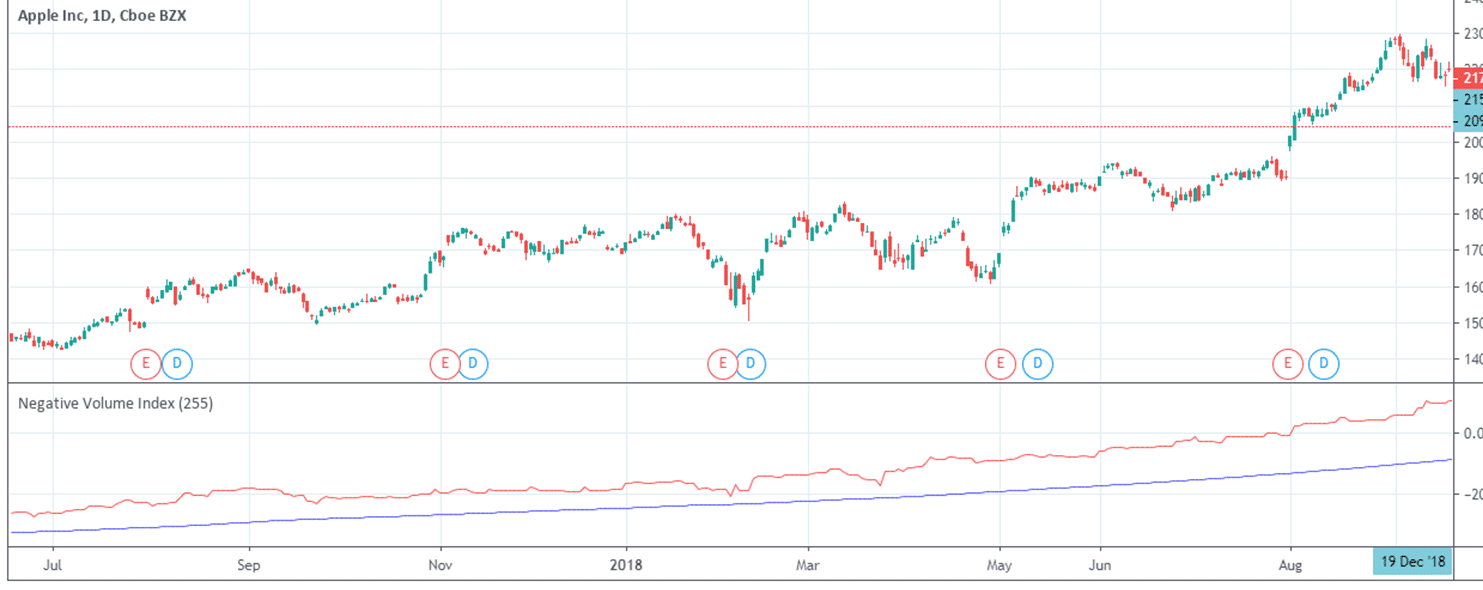Negative Volume Index

## Volume-Weighted Average Price (VWAP)

Being a volume indicator, the volume-weighted average price represents the average price an asset has traded at throughout the trading session (usually a day) when both the price and the volume are taken into account. It is the ratio of the dollar value traded to the total volume transacted over the trading period.

The VWAP gives traders some insight about the trend and value of a security. Institutional investors use the indicator to assess whether they bought at a good price for the day or have overpaid for the stock. It’s good to note that this volume indicator is plotted directly on the price chart.

### How the Volume-weighted average Price is Calculated

The VWAP is calculated by summing up the dollar value traded in the session (the average price multiplied by the number of shares transacted) and dividing by the total number traded. The formula is given as follow:

VWAP = [Sum of (Pricetypical x volume)] / sum of volume

The Calculation Involves Five Steps:

Step 1: Calculate the typical price for the chosen intraday period by finding the average of the high, low and close prices:

Typical Price = (High + Low + Close) / 3

Step 2: Multiply each period’s typical price by the volume

Step 3: Get a cumulative total of the values in step 2

Step 4: Get a cumulative total of the volume data

Step 5: Divide the values in step 3 by the value in step 4

### What Does the VWAP Tell You?

It shows the actual value the security is trading at, so it can tell if the security was bought or sold at a fair price. Stock analysts and traders use the VWAP to eliminate the noise in the market so that they can get an idea of what prices buyers and sellers are willing to transact.

When the VWAP is rising or the price crosses above the VWAP line, it shows that the price may be in an uptrend. On the other hand, if the VWAP is declining or the price crosses below the VWAP line, it suggests that the price may potentially be in a downtrend.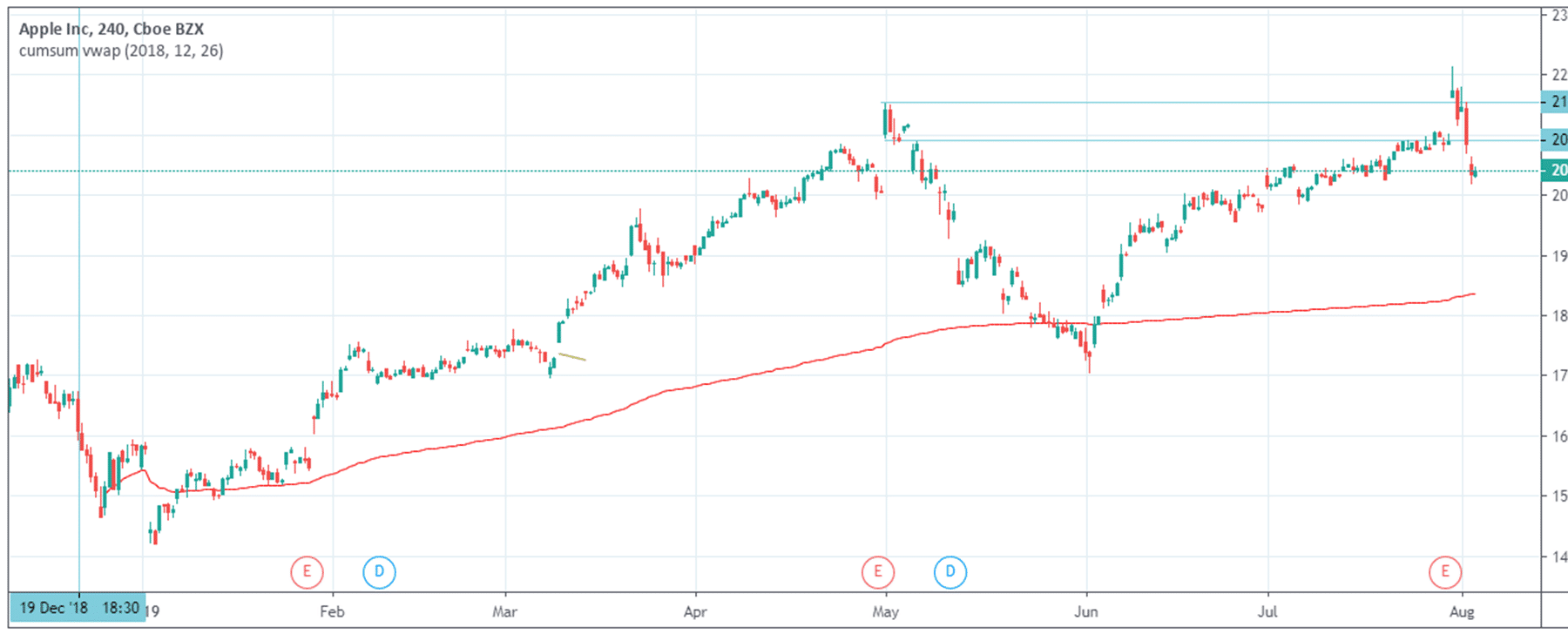Volume Weighted Average Price

## How to Use Volume and Volume Indicators in Trading

Adding one or two of these volume indicators in your analysis tools could be of great help in your trading. At the very least, a volume indicator can help you confirm the validity of a setup before you place a trade or give you an early warning about trades that aren’t progressing well.

### Breakouts and Breakdowns

In trading breakouts, most traders look for the price to close above a resistance level before placing their trades. Similarly, the price must close below a support level to be a breakdown. However, how the price closed might not be enough to confirm the validity of a breakout or breakdown.

You could add volume to the picture: a breakout or breakdown that occurs with a huge volume is more likely to succeed than one that occurs with a little volume. The reason is simple: an increase in volume during a breakout indicates strength in the move while a decrease in volume shows a lack of interest in the move.Breakout and On Balance Volume

The chart above is for Apple, and you can see the resistance line at \$196. Can you see that there’s a breakout with a gap at the beginning of August 2018? Notice the way the OBV was ascending (high volume) when the breakout occurred, indicating that there’s enough strength behind the move. See the next chart for a breakdown.Rising Volume and Breakdown

The above chart is still for Apple. You can see how the price came down to a support level, and the volume oscillator was rising when the breakdown occurred, showing the conviction of the bears. The price expectedly went down in the following sessions.

False breakouts occur frequently in the market, partly because of the liquidity it offers institutional players. Astute retail traders have learned to track this particular signature of the smart money to ride with them. In some situations, these false breakouts create patterns, such as hikkake pattern, which can be quite profitable when spotted.

Volume indicators don’t only help you to avoid trading certain breakouts that are doomed to fail but also helps you confirm whether the opposite direction presents a profitable trading setup. In other words, a volume indicator can help you identify a different trading opportunity when a breakout has failed.The chart above shows what happened to Apple in October 2018. After making a \$229.70 high in September, there was an attempt to break above the high in October, but the volume was weak, as shown by the volume oscillator lying well below the zero line. In the following days, the price turned downwards, and the volume indicator rose above the zero line, indicating the strength in the down move. That marked the beginning of a downtrend that lasted till the end of the year.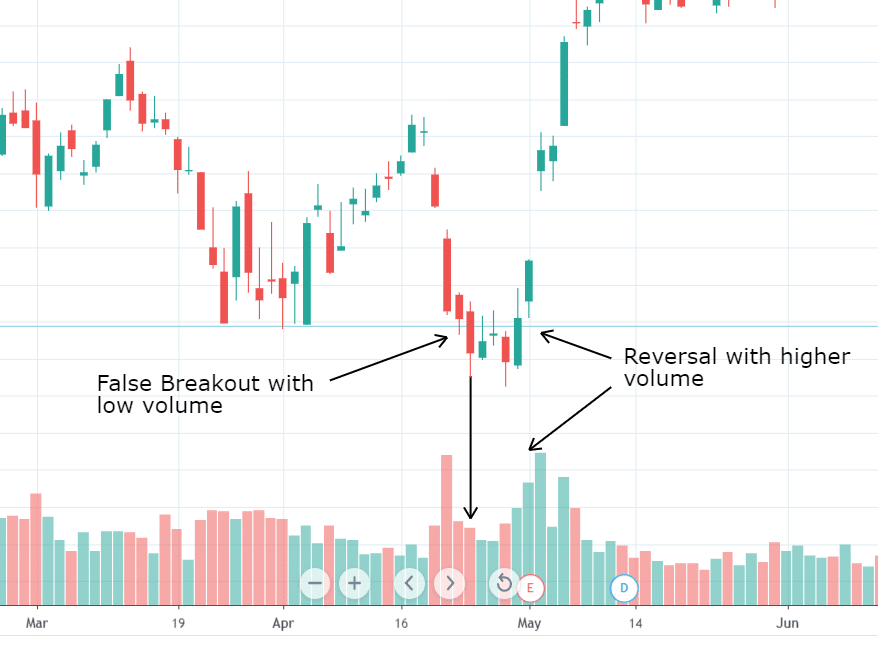False Breakout

The chart above shows Apple in the ending of April 2018. You can see the low volume during the attempted breakdown and how in the subsequent sessions, the price turned upwards with a surge in volume (arrows). This was the beginning of the upswing that lasted until October.

### Pullbacks

The price of any other security moves in waves: an impulse wave and a corrective wave (pullback). When the market is trending up or down, the impulse wave (in the direction of the trend) is larger than the corrective wave (pullback in the opposite direction). But it’s difficult to tell at the beginning of the opposite move if it’s just a correction or a price reversal.

This is where a volume indicator can help you assess what is happening and how to manage your positions. Moves in the direction of the trend are accompanied by relatively higher volumes than moves in the opposite direction. Additionally, a volume indicator can help you recognize when a pullback has completed and the trend resumed.Volume and Pullbacks

In the chart, you can see that there’s a downtrend (trendline and moving average). Notice how the volume thinned out when there was a pullback and how it started rising when the downtrend resumed.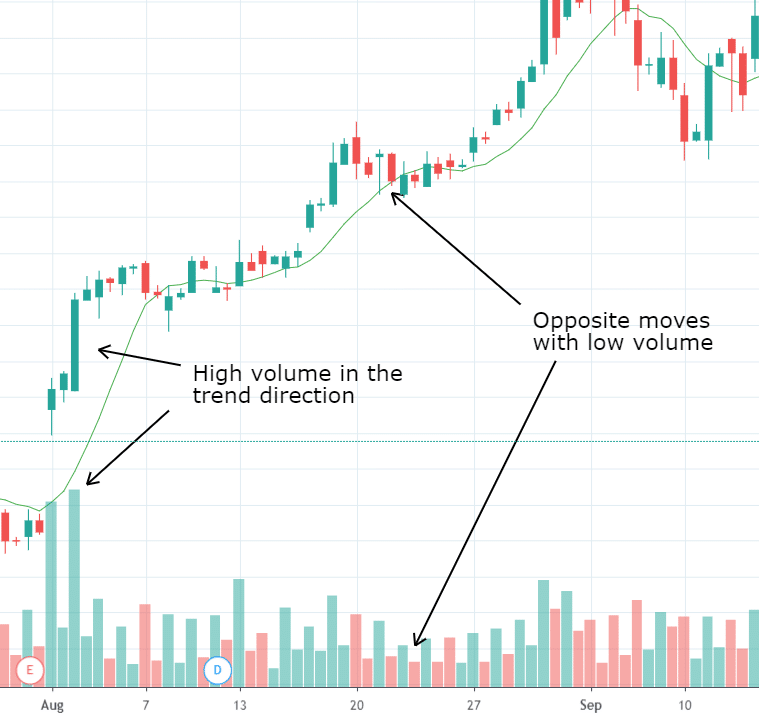Volume in rising market

The above chart shows an uptrend. Observe the huge volumes when the price is going up (first pair of arrows) and how the volume thinned out when the price pulled back (2nd set of arrows).

### Reversals

After a prolonged uptrend or downtrend, if the price begins to consolidate with relatively smaller price movements but on heavy volume, there may be a price reversal in sight. It shows that institutional investors are accumulating or distributing positions.

In addition, volume indicators can help confirm price reversal market structures, such as head and shoulder, double top, and others. The way a volume indicator behaves when the right shoulder of a head and shoulder pattern is forming can tell a lot about the setup. The same is true for a retest of the high in a double top setup. Furthermore, a divergence between the volume indicator and the price is a strong reversal signal.Bearish Volume Divergence

In the above chart, you can see a bearish divergence (Arrows), with the price making a higher high while the OBV made a lower high. It correctly predicted a downtrend.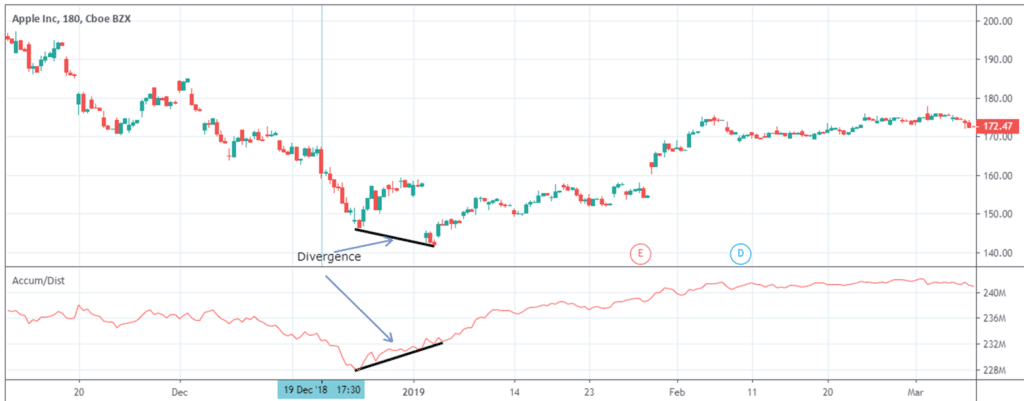Volume Divergence

The chart above shows a bullish divergence, with the price making a lower low while the A/D line was rising. What followed next was a reversal of the trend.

## Conclusion

Volume analysis is very important to traders and investors. There are numerous volume indicators out there, but we have discussed some of the commonest ones. Study them and add them to your analysis tools to improve your trading.